Next: Planetary Positions Up: Planetary Systems Previous: Planetary Systems

## Planetary Orbits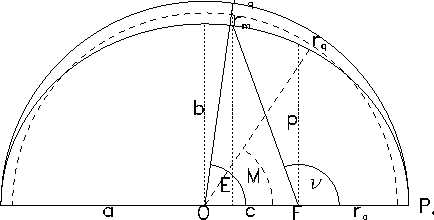As pointed out in the introduction transformations based on classical Keplerian elements can only achieve a limited precision. But for many applications it is useful to have approximate positions available. For this reason we describe in the following the calculation of position and velocity of objects in Keplerian orbits. There are many textbooks on this subject - we recommend Murray and Dermott (2000) but e.g. Bate et al. (1971),Danby (1988) or Heafner (1999) are also very useful. There are also some good web sites devoted to the subject20.

The gravitational motion of two bodies of mass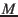and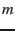and position vectors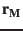and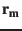can be described in terms of the three invariants: gravitational parameter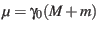, specific mechanical energy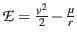, and specific angular momentum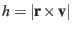, where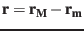,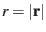and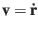.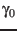is the constant of gravitation whose IAU1976 value is determined by [A. K6]: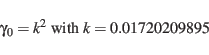(24)

when masses are given in solar masses, distances in AU [1 AU = 149 597 870 km], and times in days.

The elements of the conical orbit (shown in Fig.2) are then given as semi-major axis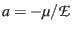and semi-minor axis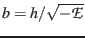, or alternatively as semi-latus rectum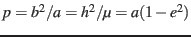and eccentricity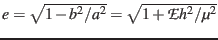. Let the origin be at the focus, the vector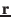then describes the motion of the body. The true anomaly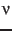is the angle betweenand the direction to the closest point of the orbit (periapsis) and can be determined from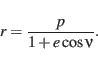(25)

If there are two focal points (ellipse, hyperbola) their distance is given by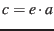, the distances of the periapsis and apoapsis are given by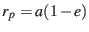and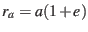. An elliptical orbit has the period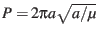.

Mean elements of a body in an elliptical orbit (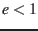) are defined by the motion of a point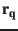on a concentric circle with constant angular velocity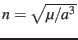and radius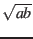, such that the orbital periodis the same forand. The mean anomaly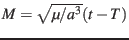is defined as the angle between periapsis and. Unfortunately there is no simple relation betweenand the true anomaly. To construct a relation one introduces another auxiliary concentric circle with radius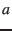and defines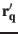as the point on that circle which has the same perifocal x-coordinate as. The eccentric anomaly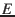is the angular distance betweenand the periapsis measured from the centre and is related to the mean and true anomalies by the set of equations: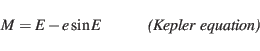(26)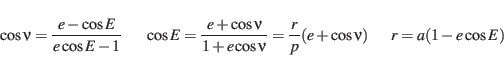(27)

Thus, if the orbital position is given as an expansion in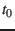of the mean longitude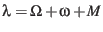, the true longitude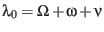can be found by an integration of the transcendental Kepler equation. In most cases a Newton-Raphson integration converges quickly (see Danby (1988) or Herrick (1971) for methods). For hyperbolic orbits (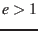) one can as well define a mean anomaly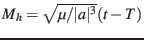but this quantity has no direct angular interpretation. The hyperbolic eccentric anomaly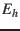is related toand the true anomalyby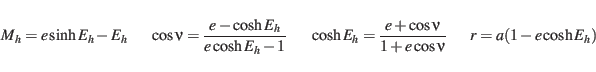(28)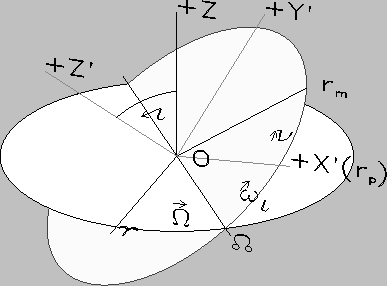The orientation of an orbit with respect to a reference plane (e.g. ecliptic) with origin at the orbital focus is defined by the inclination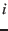of the orbital plane, the longitude of the ascending node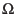, and the argument of periapsis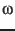which is the angle between ascending node and periapsis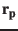(see Fig.3). The position of the body on the orbit can then be defined by its time of periapsis passage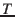, its true anomaly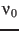at epoch, or its true longitude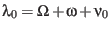at epoch. The perifocal coordinate system has its X-axis from the focus to the periapsis, and its Z-axis right-handed perpendicular to the orbital plane in the sense of orbital motion. In this system the position and velocity vector are given by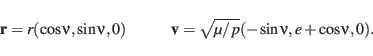(29)

These might directly be expressed by the eccentric anomaly: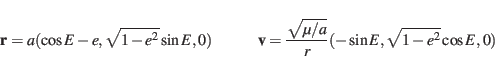(30)

In the hyperbolic case replace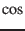byand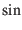by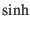. The transformation from the reference system to the perifocal system is given by the Eulerian rotation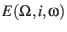as defined in the Appendix. The ecliptic position of a planet is then given by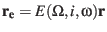.Next: Planetary Positions Up: Planetary Systems Previous: Planetary Systems
Markus Fraenz 2017-03-13